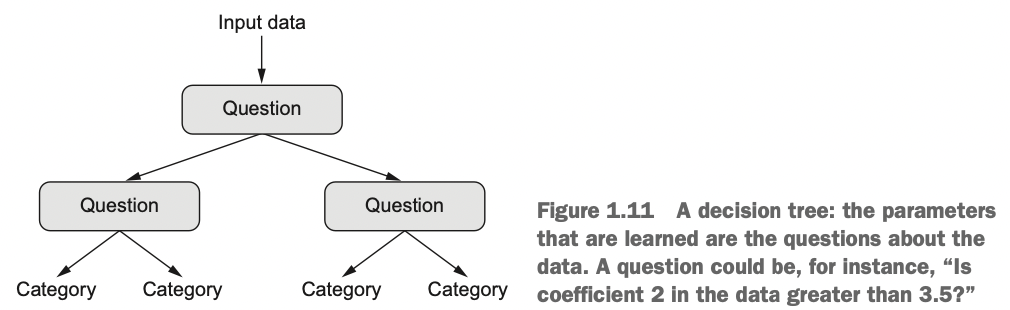# 1.2 Before deep learning: a brief history of machine learning

gabie0208·2021년 9월 17일
0

## Deep Learning with Python

목록 보기
2/3

### 1.2.1 Probabilistic modeling

Probabilistic modeling is the application of the principles of statistics to data analysis. It was one of the earliest forms of machine learning, and it’s still widely used to this day. One of the best-known algorithms in this category is the Naive Bayes algorithm.

Naive Bayes is a type of machine-learning classifier based on applying Bayes’ theo- rem while assuming that the features in the input data are all independent (a strong, or “naive” assumption, which is where the name comes from).

### 1.2.2 Early neural networks

The first successful practical application of neural nets came in 1989 from Bell Labs, when Yann LeCun combined the earlier ideas of convolutional neural networks and backpropagation, and applied them to the problem of classifying handwritten digits. The resulting network, dubbed LeNet, was used by the United States Postal Service in the 1990s to automate the reading of ZIP codes on mail envelopes.

### 1.2.3 Kernel methods

Kernel methods are a group of classification algorithms, the best known of which is the support vector machine(SVM). SVMs aim at solving classification problems by finding good decision boundaries.

SVMs proceed to find these boundaries in two steps:

• The data is mapped to a new high-dimensional representation where the decision boundary can be expressed as a hyperplane.
• A good decision boundary (a separation hyperplane) is computed by trying to maximize the distance between the hyperplane and the closest data points from each class, a step called maximizing the margin.

An SVM is a shallow method, applying an SVM to perceptual problems requires first extracting useful rep- resentations manually (a step called feature engineering), which is difficult and brittle.

### 1.2.4 Decision trees, random forests, and gradient boosting machinesKaggle (http://kaggle.com) got started in 2010, random forests quickly became a favorite on the platform—until 2014, when gradient boosting machines took over.
A gradient boosting machine, much like a random forest, is a machine-learning technique based on ensembling weak prediction models, generally decision trees.

### 1.2.5 Back to neural networks

Since 2012, deep convolutional neural networks(convnets) have become the go-to algorithm for all computer vision tasks; more generally, they work on all perceptual tasks. At major computer vision conferences in 2015 and 2016, it was nearly impossi- ble to find presentations that didn’t involve convnets in some form.
At the same time, deep learning has also found applications in many other types of problems, such as natural language processing.

### 1.2.6 What makes deep learning different

Deep learning makes problem-solving much easier, because it completely automates what used to be the most crucial step in a machine-learning workflow: feature engineering.